# Selina Solutions Concise Mathematics Class 6 Chapter 18: Fundamental Concepts Exercise 18(B)

Selina Solutions Concise Mathematics Class 6 Chapter 18 Fundamental Concepts Exercise 18(B) has detailed answers to the concepts explained under this exercise. The solved examples before each exercise, help students evaluate the type of problems that would appear in the final examination. Students can clear their doubts, without any delay, using solutions PDF, designed by subject matter experts. Those who aim to cross check their answers, can refer to Selina Solutions Concise Mathematics Class 6 Chapter 18 Fundamental Concepts Exercise 18(B) PDF, from the links which are provided, with a free download option.

## Selina Solutions Concise Mathematics Class 6 Chapter 18: Fundamental Concepts Exercise 18(B) Download PDF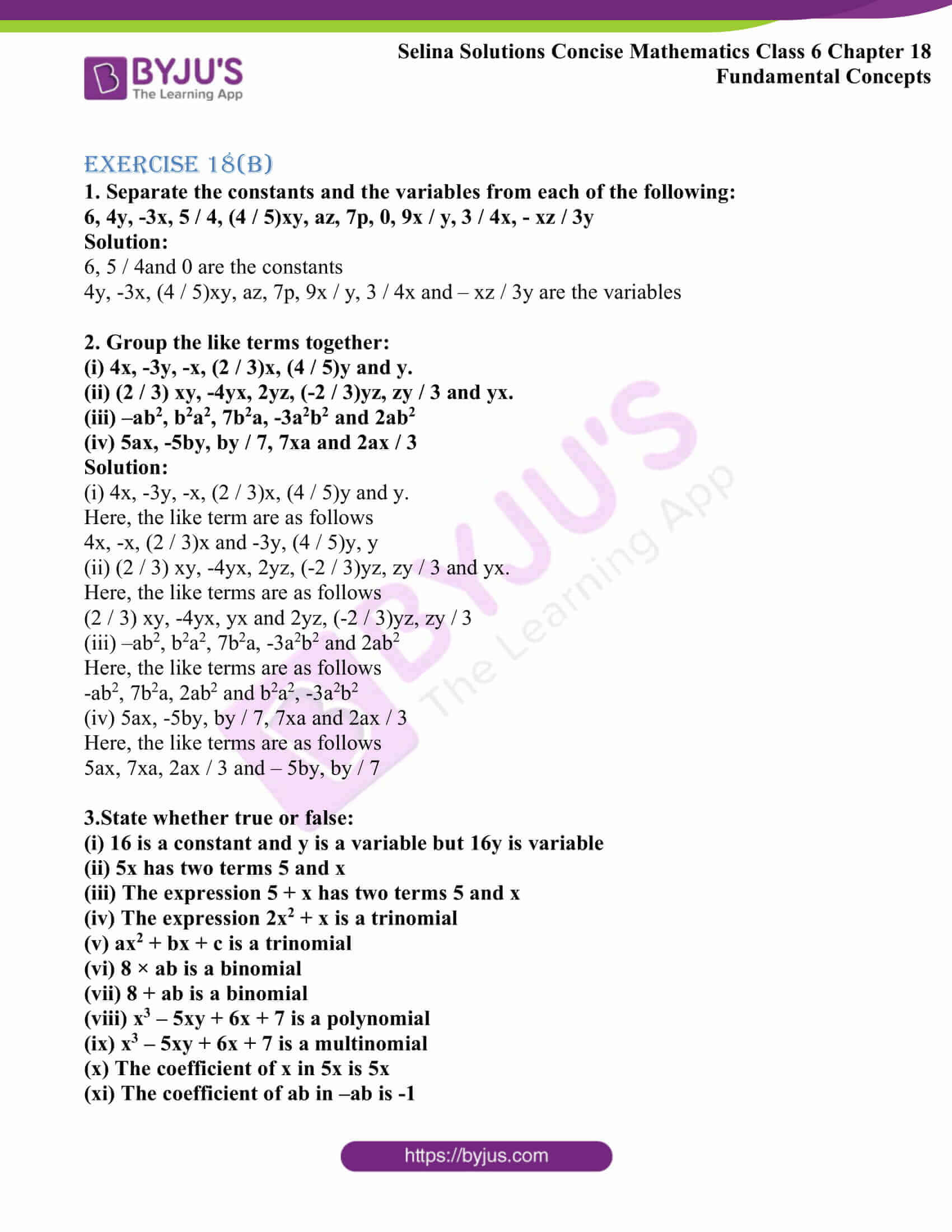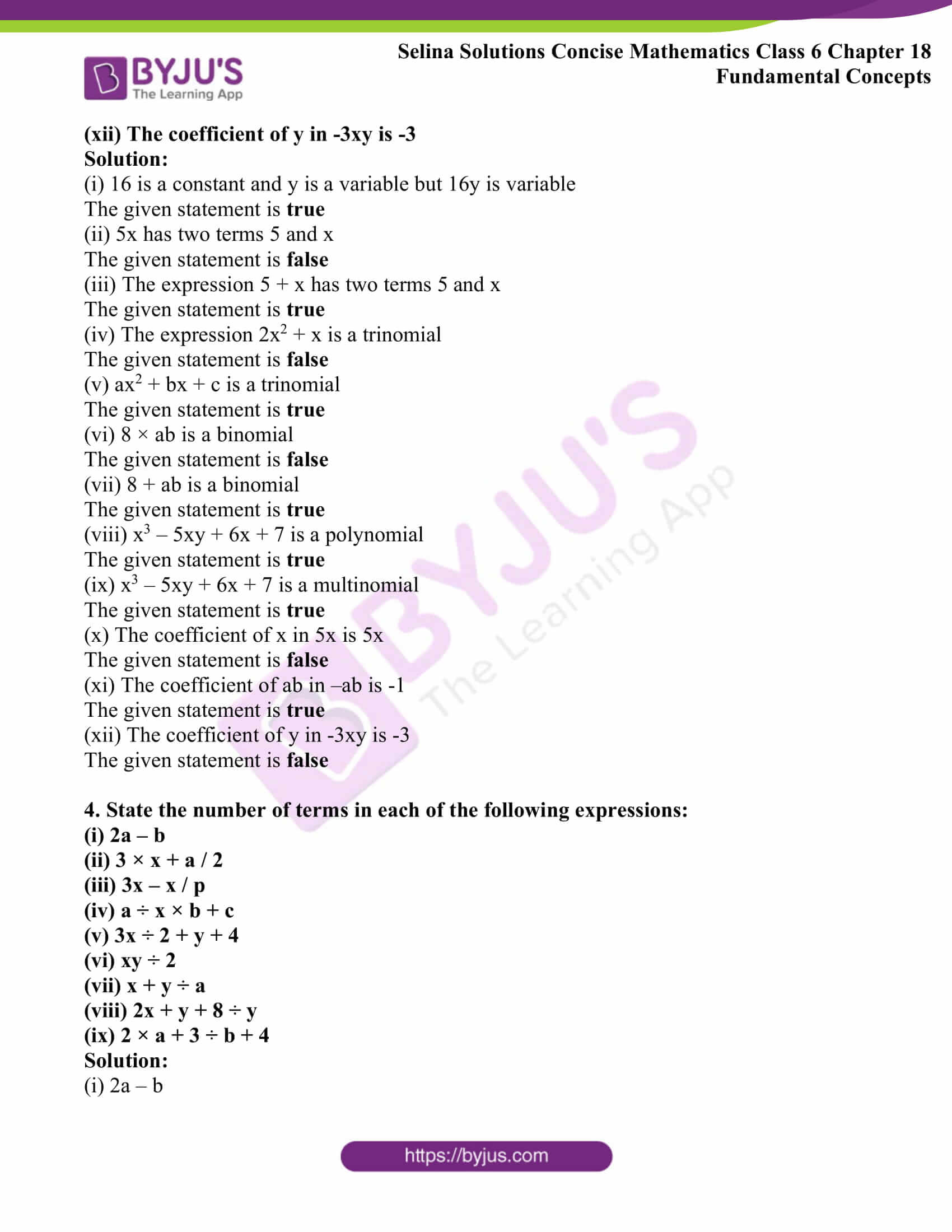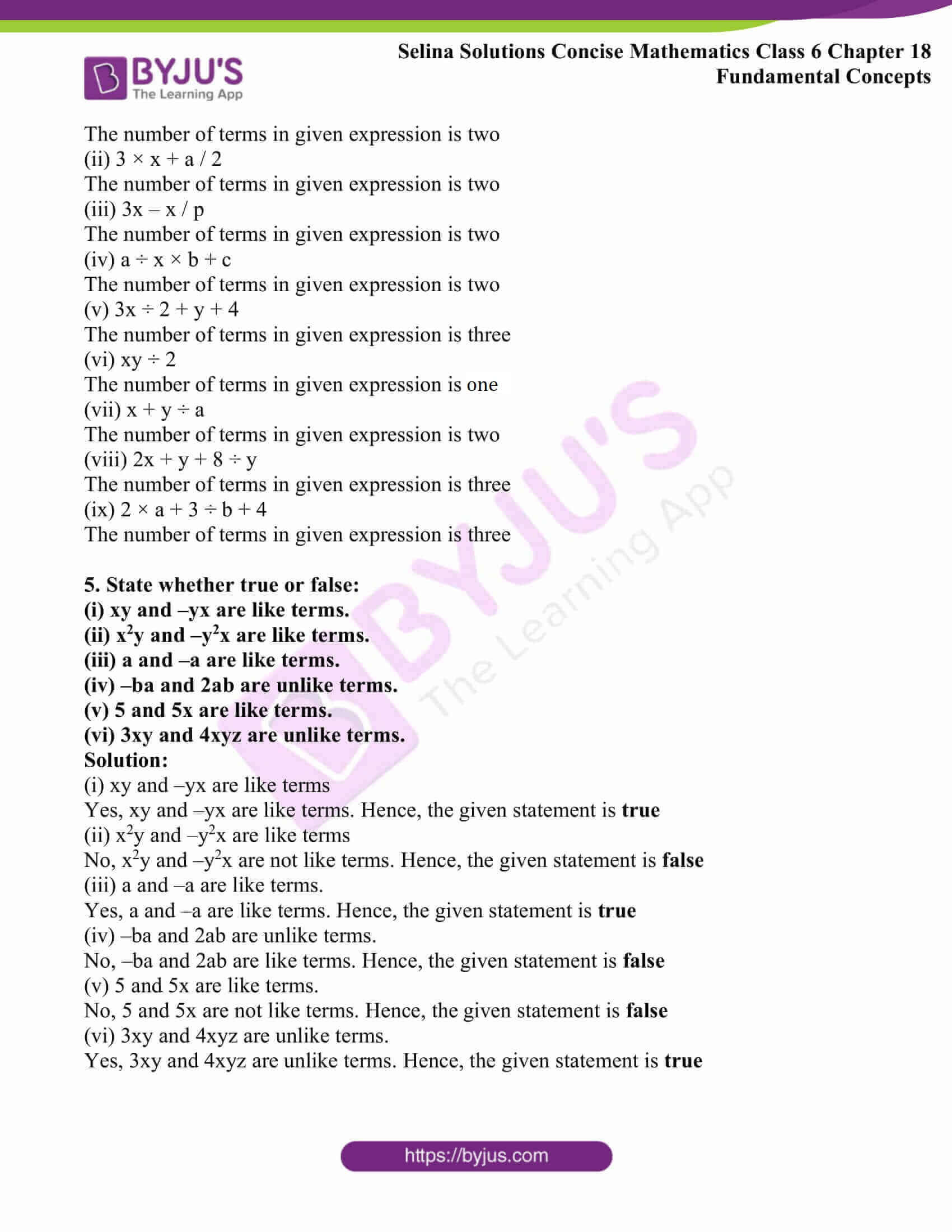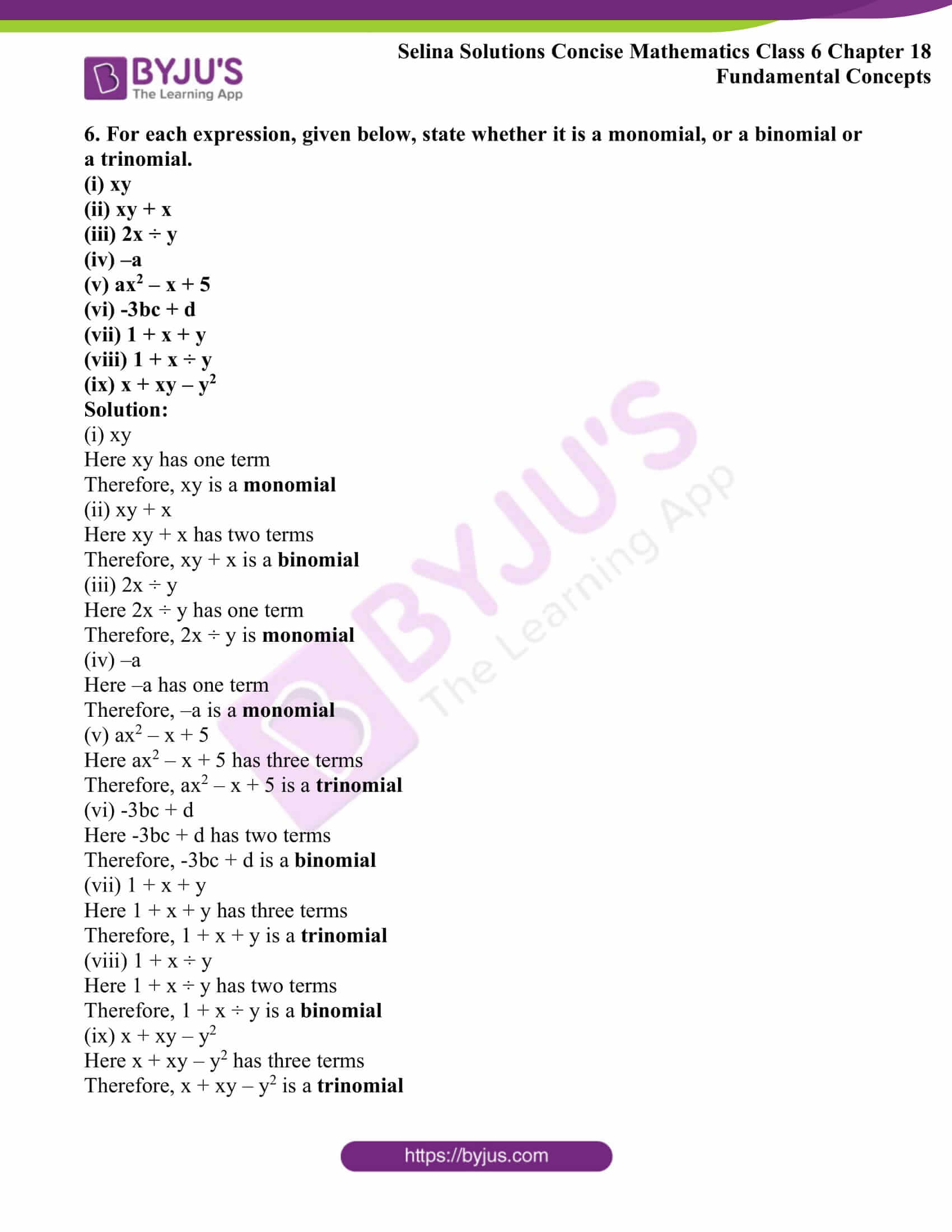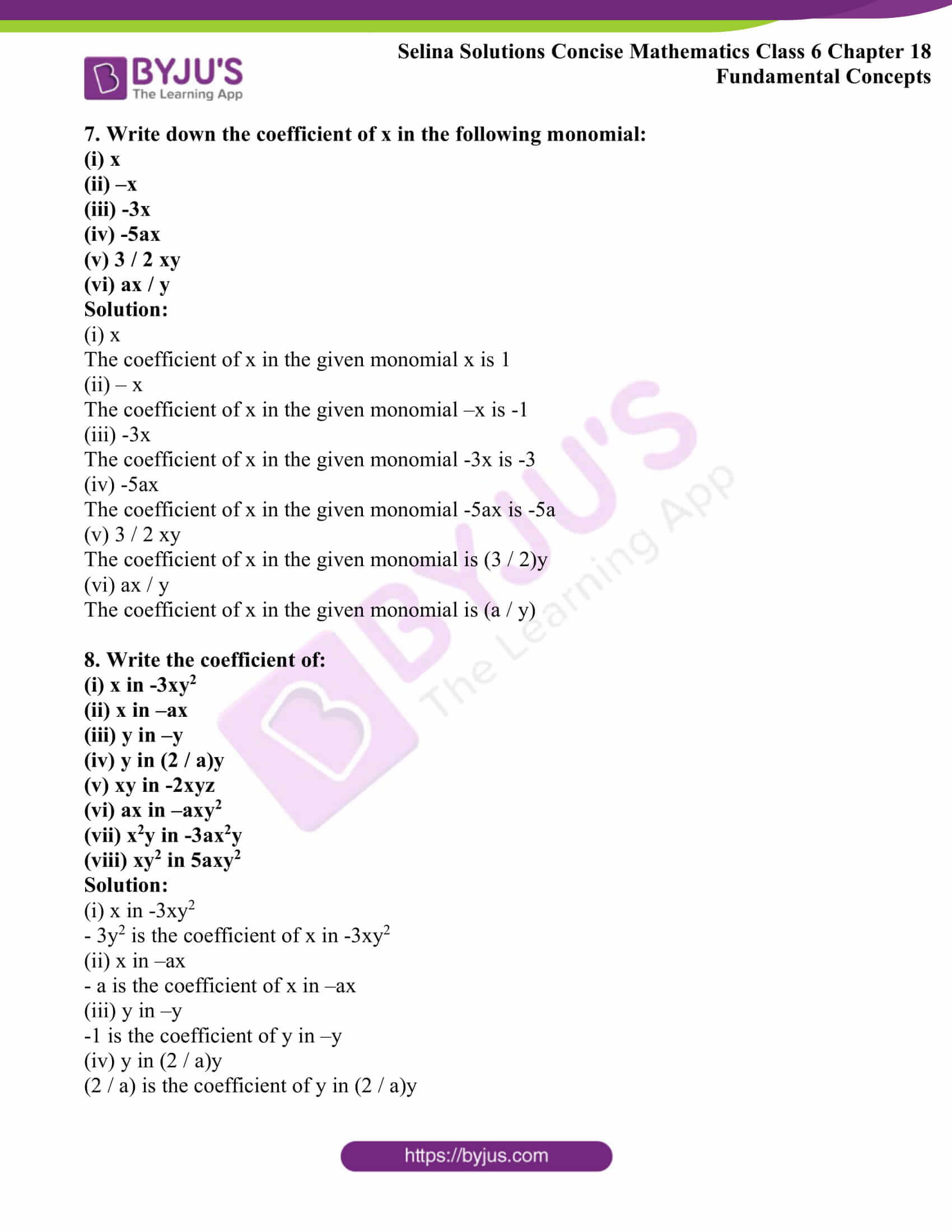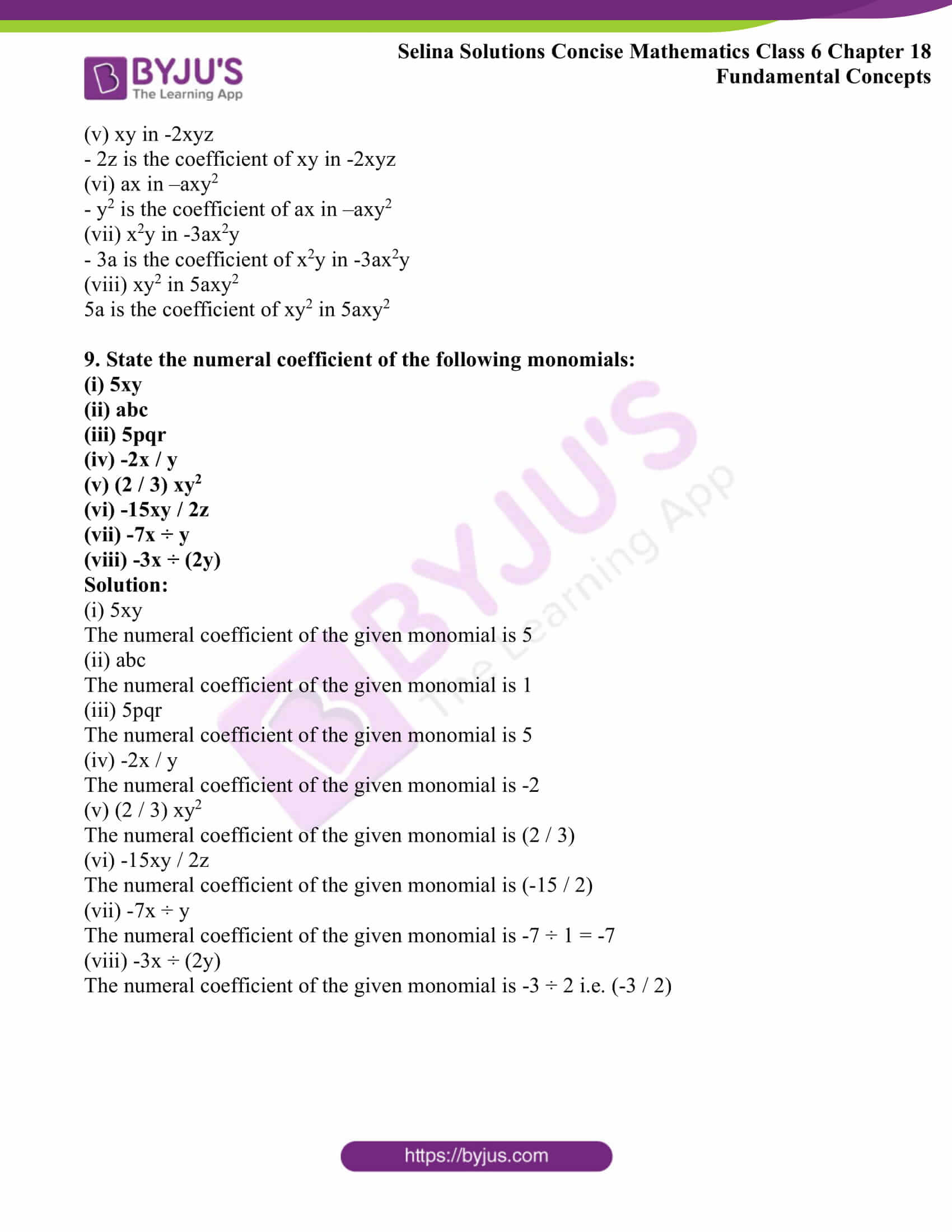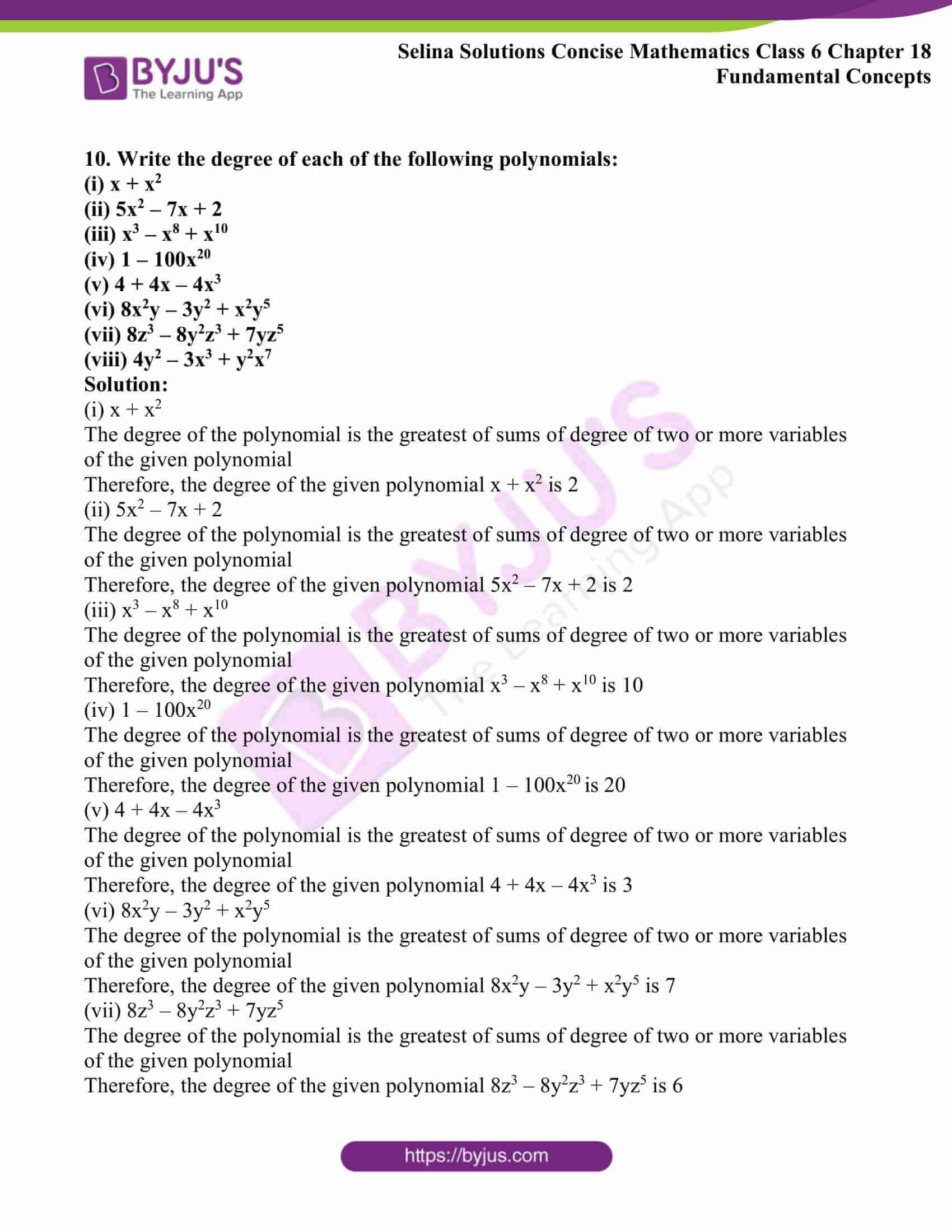### Access another exercise of Selina Solutions Concise Mathematics Class 6 Chapter 18: Fundamental Concepts

Exercise 18(A) Solutions

### Access Selina Solutions Concise Mathematics Class 6 Chapter 18: Fundamental Concepts Exercise 18(B)

Exercise 18(B)

1. Separate the constants and the variables from each of the following:

6, 4y, -3x, 5 / 4, (4 / 5)xy, az, 7p, 0, 9x / y, 3 / 4x, – xz / 3y

Solution:

6, 5 / 4and 0 are the constants

4y, -3x, (4 / 5)xy, az, 7p, 9x / y, 3 / 4x and – xz / 3y are the variables

2. Group the like terms together:

(i) 4x, -3y, -x, (2 / 3)x, (4 / 5)y and y.

(ii) (2 / 3) xy, -4yx, 2yz, (-2 / 3)yz, zy / 3 and yx.

(iii) –ab2, b2a2, 7b2a, -3a2b2 and 2ab2

(iv) 5ax, -5by, by / 7, 7xa and 2ax / 3

Solution:

(i) 4x, -3y, -x, (2 / 3)x, (4 / 5)y and y.

Here, the like term are as follows

4x, -x, (2 / 3)x and -3y, (4 / 5)y, y

(ii) (2 / 3) xy, -4yx, 2yz, (-2 / 3)yz, zy / 3 and yx.

Here, the like terms are as follows

(2 / 3) xy, -4yx, yx and 2yz, (-2 / 3)yz, zy / 3

(iii) –ab2, b2a2, 7b2a, -3a2b2 and 2ab2

Here, the like terms are as follows

-ab2, 7b2a, 2ab2 and b2a2, -3a2b2

(iv) 5ax, -5by, by / 7, 7xa and 2ax / 3

Here, the like terms are as follows

5ax, 7xa, 2ax / 3 and – 5by, by / 7

3.State whether true or false:

(i) 16 is a constant and y is a variable but 16y is variable

(ii) 5x has two terms 5 and x

(iii) The expression 5 + x has two terms 5 and x

(iv) The expression 2x2 + x is a trinomial

(v) ax2 + bx + c is a trinomial

(vi) 8 × ab is a binomial

(vii) 8 + ab is a binomial

(viii) x3 – 5xy + 6x + 7 is a polynomial

(ix) x3 – 5xy + 6x + 7 is a multinomial

(x) The coefficient of x in 5x is 5x

(xi) The coefficient of ab in –ab is -1

(xii) The coefficient of y in -3xy is -3

Solution:

(i) 16 is a constant and y is a variable but 16y is variable

The given statement is true

(ii) 5x has two terms 5 and x

The given statement is false

(iii) The expression 5 + x has two terms 5 and x

The given statement is true

(iv) The expression 2x2 + x is a trinomial

The given statement is false

(v) ax2 + bx + c is a trinomial

The given statement is true

(vi) 8 × ab is a binomial

The given statement is false

(vii) 8 + ab is a binomial

The given statement is true

(viii) x3 – 5xy + 6x + 7 is a polynomial

The given statement is true

(ix) x3 – 5xy + 6x + 7 is a multinomial

The given statement is true

(x) The coefficient of x in 5x is 5x

The given statement is false

(xi) The coefficient of ab in –ab is -1

The given statement is true

(xii) The coefficient of y in -3xy is -3

The given statement is false

4. State the number of terms in each of the following expressions:

(i) 2a – b

(ii) 3 × x + a / 2

(iii) 3x – x / p

(iv) a ÷ x × b + c

(v) 3x ÷ 2 + y + 4

(vi) xy ÷ 2

(vii) x + y ÷ a

(viii) 2x + y + 8 ÷ y

(ix) 2 × a + 3 ÷ b + 4

Solution:

(i) 2a – b

The number of terms in given expression is two

(ii) 3 × x + a / 2

The number of terms in given expression is two

(iii) 3x – x / p

The number of terms in given expression is two

(iv) a ÷ x × b + c

The number of terms in given expression is two

(v) 3x ÷ 2 + y + 4

The number of terms in given expression is three

(vi) xy ÷ 2

The number of terms in given expression is one

(vii) x + y ÷ a

The number of terms in given expression is two

(viii) 2x + y + 8 ÷ y

The number of terms in given expression is three

(ix) 2 × a + 3 ÷ b + 4

The number of terms in given expression is three

5. State whether true or false:

(i) xy and –yx are like terms.

(ii) x2y and –y2x are like terms.

(iii) a and –a are like terms.

(iv) –ba and 2ab are unlike terms.

(v) 5 and 5x are like terms.

(vi) 3xy and 4xyz are unlike terms.

Solution:

(i) xy and –yx are like terms

Yes, xy and –yx are like terms. Hence, the given statement is true

(ii) x2y and –y2x are like terms

No, x2y and –y2x are not like terms. Hence, the given statement is false

(iii) a and –a are like terms.

Yes, a and –a are like terms. Hence, the given statement is true

(iv) –ba and 2ab are unlike terms.

No, –ba and 2ab are like terms. Hence, the given statement is false

(v) 5 and 5x are like terms.

No, 5 and 5x are not like terms. Hence, the given statement is false

(vi) 3xy and 4xyz are unlike terms.

Yes, 3xy and 4xyz are unlike terms. Hence, the given statement is true

6. For each expression, given below, state whether it is a monomial, or a binomial or a trinomial.

(i) xy

(ii) xy + x

(iii) 2x ÷ y

(iv) –a

(v) ax2 – x + 5

(vi) -3bc + d

(vii) 1 + x + y

(viii) 1 + x ÷ y

(ix) x + xy – y2

Solution:

(i) xy

Here xy has one term

Therefore, xy is a monomial

(ii) xy + x

Here xy + x has two terms

Therefore, xy + x is a binomial

(iii) 2x ÷ y

Here 2x ÷ y has one term

Therefore, 2x ÷ y is monomial

(iv) –a

Here –a has one term

Therefore, –a is a monomial

(v) ax2 – x + 5

Here ax2 – x + 5 has three terms

Therefore, ax2 – x + 5 is a trinomial

(vi) -3bc + d

Here -3bc + d has two terms

Therefore, -3bc + d is a binomial

(vii) 1 + x + y

Here 1 + x + y has three terms

Therefore, 1 + x + y is a trinomial

(viii) 1 + x ÷ y

Here 1 + x ÷ y has two terms

Therefore, 1 + x ÷ y is a binomial

(ix) x + xy – y2

Here x + xy – y2 has three terms

Therefore, x + xy – y2 is a trinomial

7. Write down the coefficient of x in the following monomial:

(i) x

(ii) –x

(iii) -3x

(iv) -5ax

(v) 3 / 2 xy

(vi) ax / y

Solution:

(i) x

The coefficient of x in the given monomial x is 1

(ii) – x

The coefficient of x in the given monomial –x is -1

(iii) -3x

The coefficient of x in the given monomial -3x is -3

(iv) -5ax

The coefficient of x in the given monomial -5ax is -5a

(v) 3 / 2 xy

The coefficient of x in the given monomial is (3 / 2)y

(vi) ax / y

The coefficient of x in the given monomial is (a / y)

8. Write the coefficient of:

(i) x in -3xy2

(ii) x in –ax

(iii) y in –y

(iv) y in (2 / a)y

(v) xy in -2xyz

(vi) ax in –axy2

(vii) x2y in -3ax2y

(viii) xy2 in 5axy2

Solution:

(i) x in -3xy2

– 3y2 is the coefficient of x in -3xy2

(ii) x in –ax

– a is the coefficient of x in –ax

(iii) y in –y

-1 is the coefficient of y in –y

(iv) y in (2 / a)y

(2 / a) is the coefficient of y in (2 / a)y

(v) xy in -2xyz

– 2z is the coefficient of xy in -2xyz

(vi) ax in –axy2

– y2 is the coefficient of ax in –axy2

(vii) x2y in -3ax2y

– 3a is the coefficient of x2y in -3ax2y

(viii) xy2 in 5axy2

5a is the coefficient of xy2 in 5axy2

9. State the numeral coefficient of the following monomials:

(i) 5xy

(ii) abc

(iii) 5pqr

(iv) -2x / y

(v) (2 / 3) xy2

(vi) -15xy / 2z

(vii) -7x ÷ y

(viii) -3x ÷ (2y)

Solution:

(i) 5xy

The numeral coefficient of the given monomial is 5

(ii) abc

The numeral coefficient of the given monomial is 1

(iii) 5pqr

The numeral coefficient of the given monomial is 5

(iv) -2x / y

The numeral coefficient of the given monomial is -2

(v) (2 / 3) xy2

The numeral coefficient of the given monomial is (2 / 3)

(vi) -15xy / 2z

The numeral coefficient of the given monomial is (-15 / 2)

(vii) -7x ÷ y

The numeral coefficient of the given monomial is -7 ÷ 1 = -7

(viii) -3x ÷ (2y)

The numeral coefficient of the given monomial is -3 ÷ 2 i.e. (-3 / 2)

10. Write the degree of each of the following polynomials:

(i) x + x2

(ii) 5x2 – 7x + 2

(iii) x3 – x8 + x10

(iv) 1 – 100x20

(v) 4 + 4x – 4x3

(vi) 8x2y – 3y2 + x2y5

(vii) 8z3 – 8y2z3 + 7yz5

(viii) 4y2 – 3x3 + y2x7

Solution:

(i) x + x2

The degree of the polynomial is the greatest of sums of degree of two or more variables of the given polynomial

Therefore, the degree of the given polynomial x + x2 is 2

(ii) 5x2 – 7x + 2

The degree of the polynomial is the greatest of sums of degree of two or more variables of the given polynomial

Therefore, the degree of the given polynomial 5x2 – 7x + 2 is 2

(iii) x3 – x8 + x10

The degree of the polynomial is the greatest of sums of degree of two or more variables of the given polynomial

Therefore, the degree of the given polynomial x3 – x8 + x10 is 10

(iv) 1 – 100x20

The degree of the polynomial is the greatest of sums of degree of two or more variables of the given polynomial

Therefore, the degree of the given polynomial 1 – 100x20 is 20

(v) 4 + 4x – 4x3

The degree of the polynomial is the greatest of sums of degree of two or more variables of the given polynomial

Therefore, the degree of the given polynomial 4 + 4x – 4x3 is 3

(vi) 8x2y – 3y2 + x2y5

The degree of the polynomial is the greatest of sums of degree of two or more variables of the given polynomial

Therefore, the degree of the given polynomial 8x2y – 3y2 + x2y5 is 7

(vii) 8z3 – 8y2z3 + 7yz5

The degree of the polynomial is the greatest of sums of degree of two or more variables of the given polynomial

Therefore, the degree of the given polynomial 8z3 – 8y2z3 + 7yz5 is 6

(viii) 4y2 – 3x3 + y2x7

The degree of the polynomial is the greatest of sums of degree of two or more variables of the given polynomial

Therefore, the degree of the given polynomial 4y2 – 3x3 + y2x7 is 9